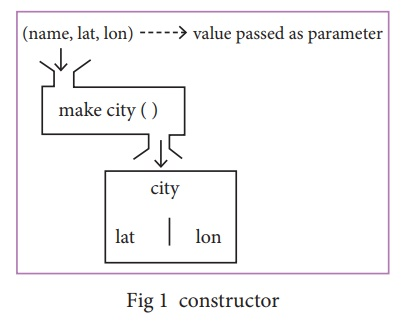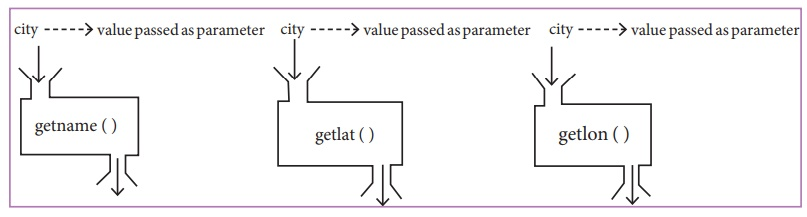Home | | Computer Science 12th Std | constructors and selectors

# constructors and selectors

Constructors are functions that build the abstract data type. Selectors are functions that retrieve information from the data type.

constructors and selectors

Constructors are functions that build the abstract data type. Selectors are functions that retrieve information from the data type.

For example, say you have an abstract data type called city. This city object will hold the city’s name, and its latitude and longitude. To create a city object, you’d use a function like

city = makecity (name, lat, lon)

To extract the information of a city object, you would use functions like

·        getname(city)

·        getlat(city)

·        getlon(city)

The following pseudo code will compute the distance between two city objects:

distance(city1, city2):

lt1, lg1 := getlat(city1), getlon(city1)

lt2, lg2 := getlat(city2), getlon(city2)

return ((lt1 - lt2)**2 + (lg1 - lg2)**2))1/2

In the above code read distance(), getlat() and getlon() as functions and read lt as latitude and lg longitude. Read := as “assigned as” or “becomes”

lt1, lg1 := getlat(city1), getlon(city1)

is read as lt1 becomes the value of getlat(city1) and lg1 becomes the value of getlont(city1).

Notice that you don’t need to know how these functions were implemented. You are assuming that someone else has defined them for us.

It’s okay if the end user doesn’t know how functions were implemented. However, the functions still have to be defined by someone.

Let us identify the constructors and selectors in the above code

As you already know that Constructors are functions that build the abstract data type. In the above pseudo code the function which creates the object of the city is the constructor.

city = makecity (name, lat, lon)

Here makecity (name, lat, lon) is the constructor which creates the object city.Selectors are nothing but the functions that retrieve information from the data type. Therefore in the above code

·        getname(city)

·        getlat(city)

·        getlon(city)

are the selectors because these functions extract the information of the city objectNow let us consider one more example to identify the constructor and selector for a slope.Read - - as comments.

- constructor

makepoint(x, y):

return x, y

- selector

xcoord(point):

return point

-selector

ycoord(point):

return point

Study Material, Lecturing Notes, Assignment, Reference, Wiki description explanation, brief detail
12th Computer Science : Chapter 2 : Data Abstraction : constructors and selectors |